# Destructive Interference

## Introduction

Only a single waveform is not sufficient to define all types of phenomena, available in nature. At a certain time, a pair of light, as well as sound waves, is found to experience interference. At the time of passing through each other, a wave front is created.

## What is interference?

In physics, interference is the net effect of two and more waves’ combinations. Two and more waves are often found to intersect within themselves. Each individual wave has a unique amplitude value (Arjunan, 2019). This value is affected by changes and frequency of interference.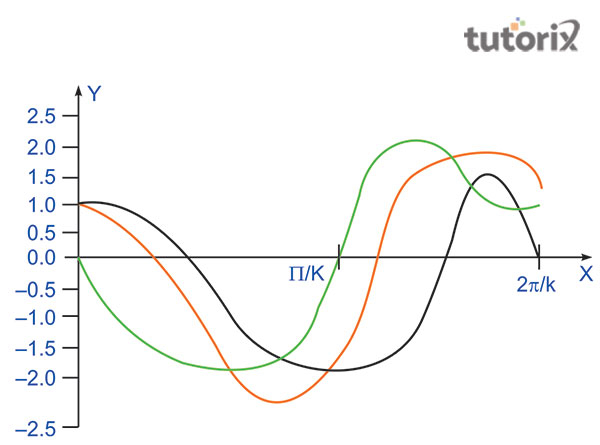Figure 1: Phase difference: δ = π/2

As presented in the above figure, the value of phase difference is “δ = π/2”. This value is equal to 90 degrees. The two thin lines interfere with respect to the resulting wave, which is presented in the red line. The red line value is equal to the sum value of the other two lines. The nodes of two individual waves are shown on the X and Y axis and this value is equal to 0.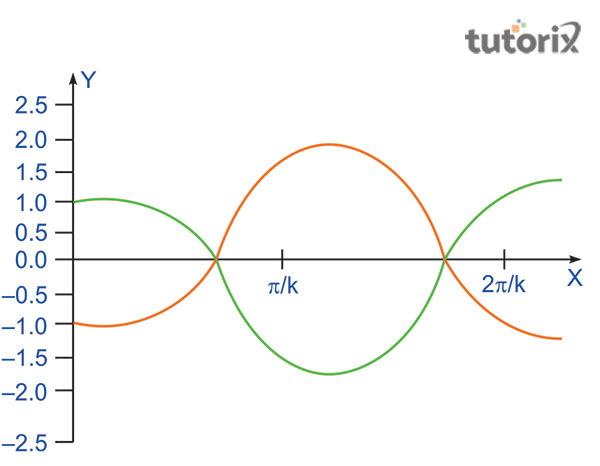Figure 2: Phase difference: δ = π

As presented above, the phase difference is “δ = π”. Two thin lines also interfere at a point, situated at the X-axis. The middle red line is the resulting wave of the other two different waves.

## Types of interference

There are main two types of wave interference, namely constructive interference and destructive interference.

• Constructive interference: This type of interference takes place at a time when two different waves are found possessing displacement and the direction of the waves are at the same point (Dittel et al. 2018). Identical frequency and its waves are included within this process. An equivalent value of amplitude superimpose is also associated with this. The sum of its intensity is very much dependent on these factors.

• Destructive interference: In a single medium, two and more two waves are travelling at some point and as a result, the total value of this wave function is algebraic total (Dittel et al. 2018). The strength of a resulting wave is often found to bear a large value. The value may possess a very small amount. The nature of destructive interference is dependent on these variations.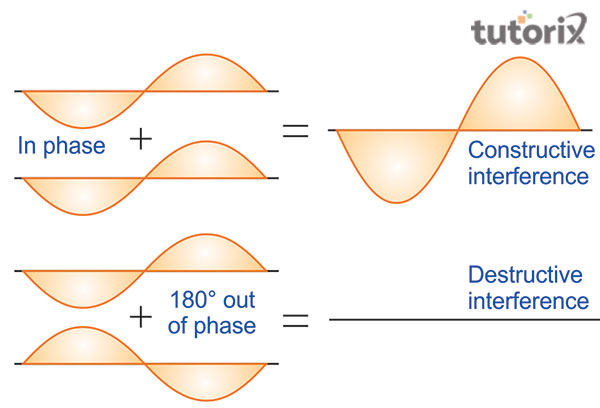Figure 3: Two types of interference

### What is Destructive interference?

At a time when the maxima of more than one wave are found at 180 degrees, destructive interference takes place. A positive displacement is responsible to cancel a negative displacement. As a result, the amplitude value becomes zero.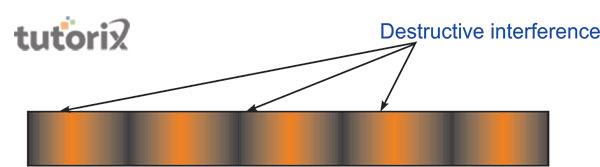Figure 4: Alternating dark and bright band, resulting from two-slit interference

In the case of interference of light waves, Young’s two-slit experiment is very significant. As per this experiment, the bands of dark lines are associated with bright lines (Miao et al. 2018). The specific dark areas are responsible and occur at the time when the waves destructively interfere with each other.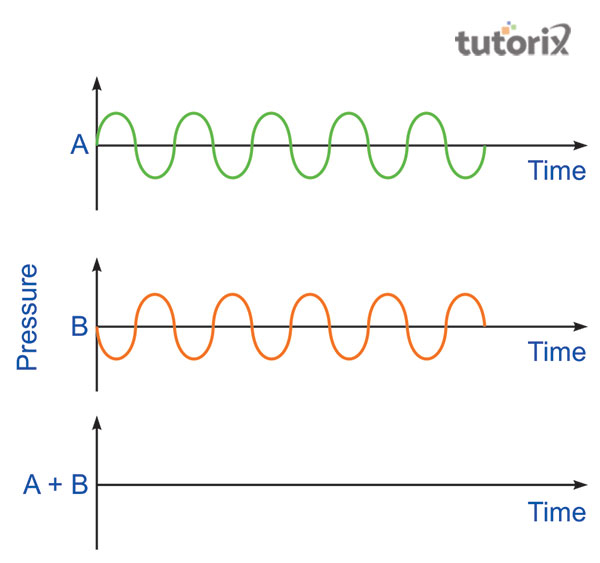Figure 5: Waves form a destructive interference

In the above-presented figure, A and B are two different waves. Both of these waves possess similar frequencies. While travelling through a medium, the values of these waves interfere. The direction of these waves is also at the same point. Two and more than two forms are found to travel in a different medium with different frequencies. The value of a resultant wave is dissimilar from each other. The sum value of the intensity of each wave is also an important factor that affects the overall nature of destructive interference (Li & Masouros, 2018). Superposition of the resultant waves is sometimes negligible that resulting in destructive interference.

### Destructive Interference Equation

• The equation with which the value of phase difference within two different waves can be presented is presented as:

$$\mathrm{(2n-1)\pi }$$

• The equation, used in presenting the difference between two individual paths that are used to present the value of two different waves, the following formula is important.

$$\mathrm{\Delta = (2n- 1)\lambda /2}$$

• The value of time that travels among two different waves is important to understand the odd multiple values. This is presented with T/2 and the formula is as follows.

$$\mathrm{\theta = (2n-1)T/2}$$

### Examples of Destructive Interference

An important destructive interference example is the gravitational waves. These kinds of waves interfere with each other at some time which creates destructive interference. Light beams are also another important example of destructive interference (Zhang et al. 2020). Radio waves and moving electrons also present the principles of destructive interference.

## Conclusion

At a time, when two and more than one wave interferes by coming close, each individual effect is added together. The highest part of the wave is a part of the crests that helps in creating the height of the original crest. Constructive interference, being the opposite nature of destructive interference possesses a significant part. In constructive interference, two waves collide and are aligned into a new wave. This wave is bigger than the original one.

## FAQs

Q1. What is the principle of superposition?

Ans. Superposition is based on the principle that states about two and more waves, traveling through a medium. The function of a resulting wave is algebraic in nature and the value is equal to the total of an individual wave function.

Q2. How the radio waves and electrons are internally connected to the destructive interference?

Ans. Moving electrons can easily undergo destructive interference. Not only that, but electrons also possess the ability to follow the principles of destructive interference.

Q3. What is the most significant example of destructive interference in real life?

Ans. In real life, the modern electronic components of an automobile muffler are a great example. As a result of destructive interference, the sounds are found to interfere in a destructive manner.

Q4. What is the amplitude value of a wave?

Ans. The amplitude value of a resulting wave is zero. This is an important part of the concept of destructive interference.

Updated on: 22-Aug-2023

172 Views